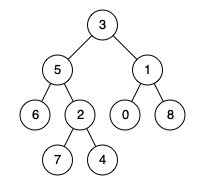## Given problem

Given a binary tree, find the lowest common ancestor (LCA) of two given nodes in the tree.

According to the definition of LCA on Wikipedia: “The lowest common ancestor is defined between two nodes p and q as the lowest node in T that has both p and q as descendants (where we allow a node to be a descendant of itself).”

1. Example 1• Input: root = `[3,5,1,6,2,0,8,null,null,7,4]`, p = 5, q = 1
• Output: 3
• Explanation: The LCA of nodes 5 and 1 is 3.
2. Example 2• Input: root = `[3,5,1,6,2,0,8,null,null,7,4]`, p = 5, q = 4
• Output: 5
• Explanation: The LCA of nodes 5 and 4 is 5, since a node can be a descendant of itself according to the LCA definition.
3. Example 3

• Input: root = `[1,2]`, p = 1, q = 2
• Output: 1
4. Constraints

• The number of nodes in the tree is in the range `[2, 105]`.
• `-109 <= Node.val <= 109`.
• All Node.val are unique.
• `p != q`.
• `p` and `q` will exist in the tree.

## Brute-force Solution

In the current brute-force solution, it has two points to get:

1. The LCA node will have the same depth from the input nodes p and q.
2. Define additional parent nodes to support get back to the root path.

So the TreeNode data structure will have additional two fields: `parent` and `depth`.

Below is the source code of this solution:

``````public TreeNode lowestCommonAncestor(TreeNode root, TreeNode p, TreeNode q) {
this.preprocessTree(root, new TreeNode(Integer.MIN_VALUE));
return this.lca(p, q);
}

/**
* Calculation of the depth and the parent of each node
*
* @param root
*/
private void preprocessTree(TreeNode root, TreeNode parent) {
if (root == null) {
return;
}

root.depth = parent.depth + 1;
root.parent = parent;

preprocessTree(root.left, root);
preprocessTree(root.right, root);
}

/**
* Find the Lowest Common Ancestor Node of a tree
*
* @param p
* @param q
* @return
*/
private TreeNode lca(TreeNode p, TreeNode q) {
Objects.requireNonNull(p);
Objects.requireNonNull(q);

while (p.depth != q.depth) {
if (p.depth > q.depth) {
p = p.parent;
} else {
q = q.parent;
}
}

while (p != q) {
p = p.parent;
q = q.parent;
}

return p;
}

private TreeNode findNode(TreeNode root, int value) {
if (root == null) {
return null;
}

if (root.val == value) {
return root;
}

TreeNode tmp1 = findNode(root.left, value);
TreeNode tmp2 = findNode(root.right, value);

return tmp1 != null ? tmp1 : tmp2;
}

public static void main(String[] args) {
LowestCommonAncestor lca = new LowestCommonAncestor();
TreeNode root = buildExample1();
TreeNode p = lca.findNode(root, 5);
TreeNode q = lca.findNode(root, 4);

TreeNode res = lca.lowestCommonAncestor(root, p, q);
System.out.println("The LCA: " + res.val);
}
``````

The complexity of this solution:

• Time complexity: O(n)
• Space complexity: O(n)

## Optimized Solution 1

In this solution, we still use DFS to iterate all Tree’s nodes. But there are two things to note here:

1. If we found the LCA node from both left and right sub-trees, we will return its parent node.
2. Otherwise, we will return one of the LCA node of the left and right sub-trees when one of them is not equal to null.

Below is our Java source code for this solution:

``````public TreeNode lowestCommonAncestor(TreeNode root, TreeNode p, TreeNode q) {
if (root == null) {
return null;
}

if (root.val == p.val || root.val == q.val) {
return root;
}

TreeNode leftLcaNode = lowestCommonAncestor(root.left, p, q);
TreeNode rightLcaNode = lowestCommonAncestor(root.right, p, q);

if (leftLcaNode != null && rightLcaNode != null) {
return root;
}

return leftLcaNode != null ? leftLcaNode : rightLcaNode;
}
``````

The complexity of this solution:

• Time complexity: O(n) with n is the number of nodes.

The maximum number of node is 10^5. By default, we will consider a computer runs 10^8 operations per second.

So the time of this solution: 10^5 / 10^8 = 10^-3 = 1ms.

• Space complexity: O(n) with n is the number of nodes because we need to maintain these nodes in recursive functions.

## Wrapping up

Refer:

236. Lowest Common Ancestor of a Binary Tree